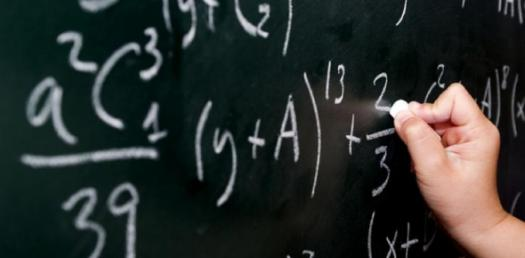# A Fundamentals Of Maths Quiz!

15 Questions | Attempts: 451300
ShareSettingsAre you preparing for a math quiz and are now looking for some revision material. The exam below is designed to help you get the highest grade you can get. Give it a try and get to know which problems you need to work more on. All the best of luck!

• 1.
What is an object measured in three dimensions of length, width, and height?
• A.

One-Dimensional.

• B.

Five-Dimensional.

• C.

Three-Dimensional.

• D.

Two-Dimensional.

• 2.
What is a polygon with four sides called?
• A.

Triangle.

• B.

Circle.

• C.

• D.

Pentagon.

• 3.
What is the amount left over when a number can't be divided equally?
• A.

Divisor.

• B.

Whole Number.

• C.

Remainder.

• D.

Odd Number.

• 4.
How many sides of the same length does a rhombus have?
• A.

Three.

• B.

Eighteen.

• C.

One.

• D.

Four.

• 5.
What is the part of a line that has an endpoint and runs in one direction?
• A.

Center.

• B.

Ray.

• C.

Mid-line.

• D.

• 6.
What kind of clock has hands moving on it for showing hours and minutes?
• A.

Stopwatch.

• B.

Sundial.

• C.

Digital.

• D.

Analog.

• 7.
What is 10 - 4 equal to?
• A.

2

• B.

10

• C.

19

• D.

6

• 8.
What is the line segment where two faces of a solid figure meet?
• A.

Surface.

• B.

Vertex.

• C.

Diameter.

• D.

Edge.

• 9.
In which direction(s) does a plane extend?
• A.

Right To Left Only.

• B.

In All Directions.

• C.

Left To Right Only.

• D.

Up And Down Only.

• 10.
What is a "point"?
• A.

An Estimation.

• B.

Another Word For Sum.

• C.

A Factor In A Sentence.

• D.

An Exact Location In Space.

• 11.
Which unit is used to measure angles or temperatures?
• A.

Inch.

• B.

Decimeter.

• C.

Degree.

• D.

Point.

• 12.
If two objects have the same shape, but are different in size, then they are what?
• A.

Even.

• B.

Prime.

• C.

Similar.

• D.

Odd.

• 13.
How many right angles does a square have?
• A.

4

• B.

1

• C.

24

• D.

26

• 14.
In an expression, what is missing?
• A.

Minus Signs.

• B.

An Equal Sign.

• C.

• D.

The Number Three.

• 15.
Which triangle has three congruent sides?
• A.

Uneven.

• B.

Double.

• C.

Equilateral.

• D.

Right.

## Related TopicsBack to top
×

Wait!
Here's an interesting quiz for you.